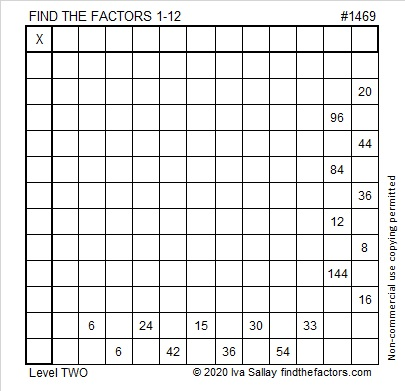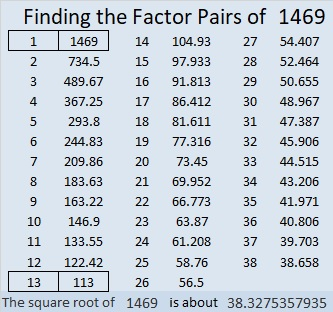# 1469 and Level 2

Contents

### Today’s Puzzle:

Where do the Factors 1-12 belong on this multiplication table puzzle?### Factors of 1469:

• 1469 is a composite number.
• Prime factorization: 1469 = 13 × 113
• 1469 has no exponents greater than 1 in its prime factorization, so √1469 cannot be simplified.
• The exponents in the prime factorization are 1, and 1. Adding one to each exponent and multiplying we get (1 + 1)(1 + 1) = 2 × 2 = 4. Therefore 1469 has exactly 4 factors.
• The factors of 1469 are outlined with their factor pair partners in the graphic below.### Other Facts about the Number 1469:

1469 is the sum of two squares in two different ways:
38² + 5² = 1469
37² + 10² = 1469

1469 is the hypotenuse of FOUR Pythagorean triples:
195-1456-1469 which is 13 times (15-112-113)
380-1419-1469 calculated from 2(38)(5), 38² – 5², 38² + 5²
565-1356-1469 which is (5-12-13) times 113
740-1269-1469 calculated from 2(37)(10), 37² – 10², 37² + 10²

This site uses Akismet to reduce spam. Learn how your comment data is processed.